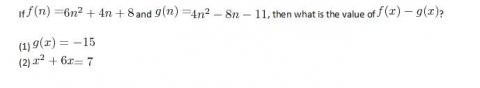# GMAT 800 Data Sufficiency

 Categories : GMAT 800, GMAT 800 Data SufficiencyA.    Statement (1) ALONE is sufficient, but statement (2) alone is not sufficient.B.    Statement (2) ALONE is sufficient, but statement (1) alone is not sufficient.C.    BOTH statements TOGETHER are sufficient, but NEITHER statement ALONE is sufficient.D.    EACH statement ALONE is sufficient.E.    Statements (1) and (2) TOGETHER are NOT sufficient to answer the question asked, and additional data are needed.Explanation:This question asks whether the information in one or both statements is sufficient to find a specific value. But we don't actually have to determine the value, just know that it can be determined.(1) Quadratic equations have two solutions. However, sometimes those two solutions are identical with each other. If they are different, then solving this equation will lead to two possible values of x, and presumably also two possible values of f(x), so this would not be sufficient. But if they are the same, i.e. if there's really only one solution to this equation, then we would definitely know x, definitely know f(x), and... Categories : GMAT 800 Data Sufficiency, Exponents If n and a are positive integers, what is the units digit of n^(4a+2) – n^(8a)?(1) n = 3(2) a is oddA) Statement (1) ALONE is sufficient, but statement (2) alone is not sufficient to answer the question asked;B)    Statement (2) ALONE is sufficient, but statement (1) alone is not sufficient to answer the question asked;C)    BOTH statements (1) and (2) TOGETHER are sufficient to answer the question asked, but NEITHER statement ALONE is sufficient;D)    EACH statement ALONE is sufficient to answer the question asked;E)    Statements (1) and (2) TOGETHER are NOT sufficient to answer the question asked, and additional data are needed.BONUS QUESTION: What actually is the units digit (assuming the answer is not E)?ANSWER BELOWOne important thing to note about exponents is that, by definition, they indicate “repetitive multiplication” – the multiplication of the same number over and over again.  Accordingly, they lend themselves nicely to patterns, as when you perform the  same action over and over again you’ll tend to get similar results.  When you consider statement 1, that n = 3, look at how 3 multiplies to different exponents:...

Get F1GMAT's Newsletters (Best in the Industry)

• Ranking Analysis
• Post-MBA Salary Trends
• Post-MBA Job Function & Industry Analysis
• Post-MBA City Review
• MBA Application Essay Tips
• School Specific Essay Tips
• GMAT Preparation Tips